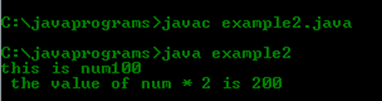## Write a simple java program to display a string and perform a simple multiplication.

Category : Java | Sub Category : The Genesis of Java | By Runner Dev Last updated: 2020-09-27 11:49:51 Viewed : 251

Write a simple java program to display a string and perform a simple multiplication.

DescriptionIn this program , Create a class with name of example2, after this we initialize num and give the value and show value of num in form of output,after this we declare ―num = num * 2‖And than show thevalue of num after multiplying by 2.

Code:

/**

*

* . Write a simple java program to display a string and perform a   simple  multiplication.

*/

public class Example_2 { // This line daclare a new class & the class defination is start.

public static void main(String a[]) { // This line begins the main() method.

int num; // this declares a variable called num.

num = 100; // this assign num the value 100.

System.out.println("this is num: " + num);

// this line show output of num //This line outputs the string and number.

num = num * 2; // this line show value of num is equals to num multiply by 2

System.out.print("The value of num * 2 is "); // This line outputs the string "The value of num * 2 is "

System.out.println(num); // this is a output line which give the value of num

//This line output the value store in variable num followed by a new line.

} // end of main prograam

// end of class

Output: